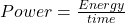## A 100-watt lightbulb uses 1 kilowatt-hour of electrical energy when it is on for ten hours. How much energy does a 25-watt light

Question

A 100-watt lightbulb uses 1 kilowatt-hour of electrical
energy when it is on for ten hours. How much energy does
a 25-watt lightbulb use when it is on for ten hours?

in progress 0
5 months 2021-08-31T06:03:42+00:00 1 Answers 1 views 0

Energy = 0.25 kilowatt-hour

Explanation:

Given the following data;

Power = 25 Watts

Time = 10 hours

Power can be defined as the energy required to do work per unit time.

Mathematically, it is given by the formula;To find the energy consumed;

Energy = power * time

Substituting into the formula, we have;

Energy = 25 * 10

Energy = 250 Watt-hour

To convert to kilowatt-hour, we would divide by 1000;

Energy = 250/1000

Energy = 0.25 kilowatt-hour